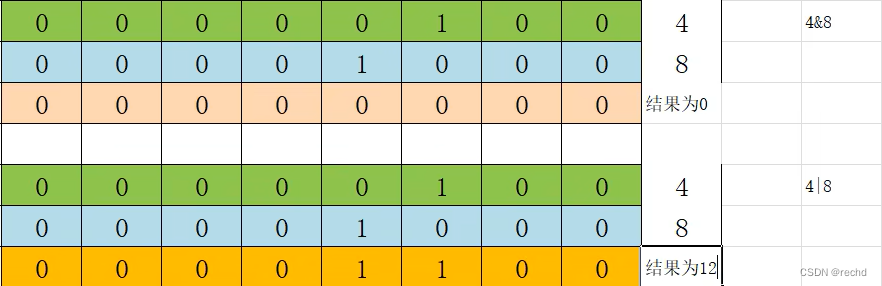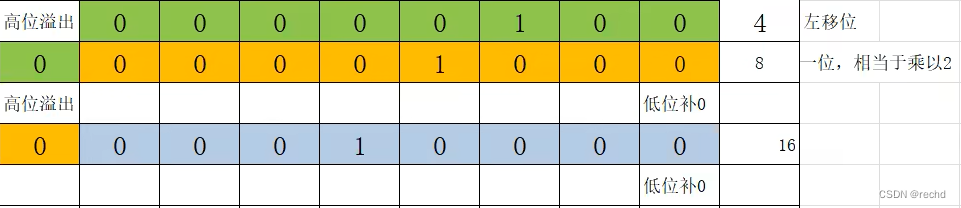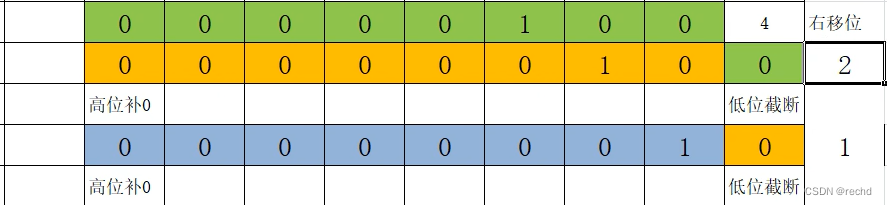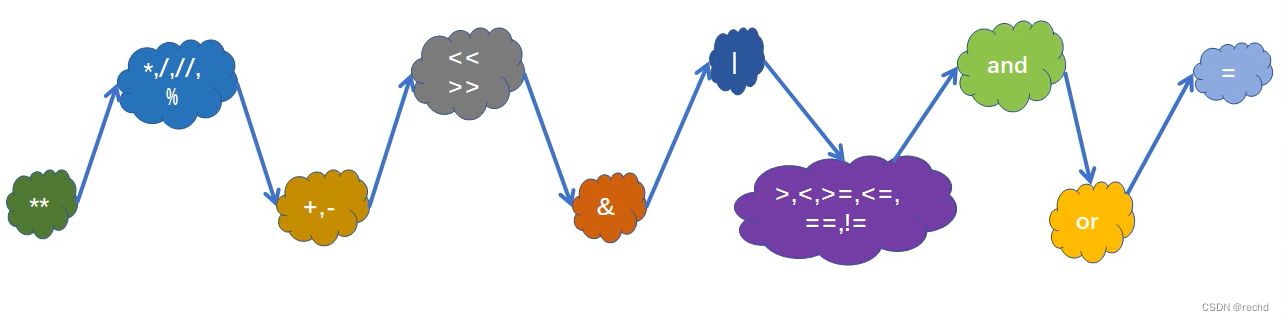### python编程入门总结1

• 输入输出函数
• print输出
• input输入
• 转义字符与注释
• 转义字符
• 注释
• 变量
• 命名规则
• 变量的结构
• 类型
• 整型
• 浮点型
• 布尔类型
• 字符串类型
• 类型转换
• 运算符
• 算数运算符
• 赋值运算符
• 比较运算符
• 布尔运算符
• 位运算符
• 运算符的优先级
• 程序的结构
• 对象的布尔值
• 选择结构
• 内置函数range()
• 循环结构
• 流程控制语句
• else语句
• 循环嵌套
• 二层循环中break和continue

# 输入输出函数

## print输出

``````print(520)
print('helloword')
print(23*25)
``````
• 1
• 2
• 3

``````fp=open('D:/text.txt','a+')#如果文件不存在就创建，如果存在就追加
print('hello',file=fp)
fp.close()
``````
• 1
• 2
• 3

``````print('hello','world','python')# hello world python
``````
• 1

## input输入

input函数接收输入数据默认为字符串类型str。

``````present=input('请输入：')#括号内字符串为输入前的提示信息。
print(present,type(present))#这里的present变量类型是str。input函数输入的默认格式就是str。
``````
• 1
• 2

input使用：加法计算两个的输入数字

``````a=input('输入一个加数')
b=input('输入另一个加数')
print(int(a)+int(b))#由于input接受类型为str，所以这里需要进行类型转换。否则就是字符串的拼接。
``````
• 1
• 2
• 3

# 转义字符与注释

## 转义字符

\n 换行
\t 制表位
\b backspace退一格/删除
\r return回车

``````print('\'nihao\'')# 'nihao'
``````
• 1

# 变量

## 命名规则

• 字母、数字、下划线
• 不能以数字开头
• 不能是保留字
• 严格区分大小写

``````import keyword
print(keyword.kwlist)
#['False', 'None', 'True', 'and', 'as', 'assert', 'break', 'class', 'continue', 'def', 'del', 'elif', 'else', 'except', 'finally', 'for', 'from', 'global', 'if', 'import', 'in', 'is', 'lambda', 'nonlocal', 'not', 'or', 'pass', 'raise', 'return', 'try', 'while', 'with', 'yield']
``````
• 1
• 2
• 3

## 变量的结构

• 标识：表示对象所存储的内存地址，使用内置函数id(obj)来获取。
• 类型：表示对象的数据类型，使用内置函数type(obj)来获取。
• 值：表示对象所存储的具体数据，使用print(obj)可以将值进行打印输出。
``````name='李四'
print(name)
print(id(name))#获取对象在内存当中的地址(标识)
print(type(name))#获取对象数据类型
name='张三'#同一变量被多次赋值，name将指向新的内存地址，这里是存储张三的内存地址。
#存储李四的变量将会被视为内存垃圾，python垃圾回收机制会自动回收。
``````
• 1
• 2
• 3
• 4
• 5
• 6

## 类型

### 整型

• 十进制：默认的进制
• 二进制：以0b开头
• 八进制：以0o开头
• 十六进制：以0x开头
``````print(118)
print(0b10101111)
print(0o176)
print(0x1EAF)
``````
• 1
• 2
• 3
• 4

### 浮点型

``````from decimal import Decimal
n1=1.1
n2=1.2
print(n1+n2)#2.3
``````
• 1
• 2
• 3
• 4

true表示1，false表示0

## 类型转换

str() 将其他数据类型转换成字符串 也可用引号转换 str(123) ‘123’
int() 将其他类型转换成整数 1、文字类和小数字符串，无法转成整数 2、浮点数转换成整数：抹零取整 int(‘123’) int(9.8)
float() 将其他数据类型转成浮点数 1、文字类无法转成整数 2、整数转成浮点数，末尾为.0 float(‘9.9’) float(9)
``````#str()
wname='王五'
age=20
print('我是'+wname+'今年'+str(age)+'岁')
#int():只能转整数串，文字类和小数类不可。例：t1='1.23'可以先转float再转int
#float()：无法转文字类字符串，其他均可。
``````
• 1
• 2
• 3
• 4
• 5
• 6

# 运算符

## 算数运算符

``````print(1+1)#2
print(2-1)#1
print(2*2)#4
print(1/2)#0.5
print(11//2)#整除运算 5
print(11%2)#1
print(2**2)#2的2次方 4
``````
• 1
• 2
• 3
• 4
• 5
• 6
• 7
``````print(9//4)#2
print(-9//-4)#2
#异号整除，向下取整
print(9//-4)#-3
print(-9//4)#-3
#异号取余要遵循公式：余数=被除数-除数*商
print(9%-4)#9-(-4)*(-3)=-3
print(-9%4)#-9-(4)*(-3)=3
``````
• 1
• 2
• 3
• 4
• 5
• 6
• 7
• 8

## 赋值运算符

“=”是赋值运算符，执行顺序是从右到左的。

``````a=b=c=20
print(a,id(a))#20 1353999168
print(b,id(b))#20 1353999168
print(c,id(c))#20 1353999168
``````
• 1
• 2
• 3
• 4

+=、-=、*=、/=、//=、%=

``````a=20
a+=30#a=a+30
a-=10#a=a-10
a*=20#a=a*20
a/=2#a=a/2
a//=2#a=a//2
``````
• 1
• 2
• 3
• 4
• 5
• 6

a,b,c=20,30,40

``````a,b,c=20,30,40
print(a,b,c)#20 30 40
#左右个数必须对应
e,f=20,30,40#报错
#交换值
s1,s2=10,20
s1,s2=s2,s1
print(s1,s2)#20,10
``````
• 1
• 2
• 3
• 4
• 5
• 6
• 7
• 8

## 比较运算符

>、<、>= 、<= 、!=

``````a,b,c=20,30,20
print(a>=b)#False
print(a==c)#True
``````
• 1
• 2
• 3

==、is、is not
`一个“ = ”称为赋值运算符，“ == ”称为比较运算符。 一个变量由三部分组成，标识、类型、值。“ == ” 比较的是值，“ is ”比较的是标识。 `

``````a=b=10
print(a==b)#True
print(a is b)#True
print(a is not b)#False

list1=[11,22,33,44]
list2=[11,22,33,44]
print(list1==list2)#True
print(list1 is list2)#False
print(id(list1),id(list2))#2197020279368 2197020314696不等，所以为false
``````
• 1
• 2
• 3
• 4
• 5
• 6
• 7
• 8
• 9
• 10

## 布尔运算符

and

``````a,b=1,2
print(a==1 and b==2)#True
print(a==1 and b<2)#True and False -->False
print(a!=1 and b==2)#False and True -->False
print(a!=1 and b!=2)#False and False -->False
``````
• 1
• 2
• 3
• 4
• 5

or

``````a,b=1,2
print(a==1 or b==2)#True
print(a==1 or b<2)#True or False -->True
``````
• 1
• 2
• 3

not

``````f1=True
f2=False
print(not f1)#False
print(not f2)#True
``````
• 1
• 2
• 3
• 4

in、not in

``````s='helloworld'
print('w' in s)#True
print('k' in s)#False
print('w' not in s)#False
print('k' not in s)#True
``````
• 1
• 2
• 3
• 4
• 5

## 位运算符

& 、|

``````print(4&8)#0
print(4|8)#12
``````
• 1
• 2>> 、<<

``````print(4<<1)#8 向左移动1位，相当于*2
print(4<<2)#16 向左移动2位，相当于*4
print(4>>1)#2 向右移动1位，相当于/2
print(4>>2)#1 向右移动2位，相当于/4
``````
• 1
• 2
• 3
• 4## 运算符的优先级# 程序的结构

## 对象的布尔值

Python一切皆对象，所有对象都有一个布尔值——获取对象布尔值，函数bool()

• Fasle
• 数值0
• None
• 空字符串
• 空列表
• 空元组
• 空字典
• 空集合
``````#以下结果皆为False，
print(bool(False))
print(bool(0))
print(bool(0.0))
print(bool(None))
print(bool(''))
print(bool(""))
print(bool([]))#空列表
print(bool(list()))#空列表
print(bool(()))#空元组
print(bool(tuple()))#空元组
print(bool({}))#空字典
print(bool(dict()))#空字典
print(bool(set()))#空集合

#其他对象的布尔值均为True。
print(bool('aaa'))#True
print(bool(10))#True
``````
• 1
• 2
• 3
• 4
• 5
• 6
• 7
• 8
• 9
• 10
• 11
• 12
• 13
• 14
• 15
• 16
• 17
• 18

## 选择结构

``````if 条件表达式:
条件执行体
``````
• 1
• 2

``````if 条件表达式:
条件执行体1
else:
条件执行体2
``````
• 1
• 2
• 3
• 4
``````money=200
s=int(input('请输入取款金额：'))
if money>=s:
money-=s
print('取款成功，余额为',money)
else:
print('余额不足')
``````
• 1
• 2
• 3
• 4
• 5
• 6
• 7

``````if 条件表达式1:
条件执行体1
elif 条件表达式2:
条件执行体2
elif 条件表达式N：
条件执行体N
[else:]
[条件执行体N+1]
``````
• 1
• 2
• 3
• 4
• 5
• 6
• 7
• 8

``````if 条件表达式：
if 内层条件表达式:
内层条件执行体1
else:
内层条件执行体2
else:
条件执行体
``````
• 1
• 2
• 3
• 4
• 5
• 6
• 7

``````x if 判断条件 else y
``````
• 1

pass语句

``````if answer=='y'
print('OK')
else:
pass
``````
• 1
• 2
• 3
• 4

## 内置函数range()

range()函数用于生成一个整数序列，创建range对象有三种方式：

range(stop) 创建一个(0,stop)之间的整数序列，步长为1
range(start,stop) 创建一个(start,stop)之间的整数序列，步长为1
range(start,stop,step) 创建一个(start,stop)之间的整数序列，步长为step

range()的返回值是一个迭代器对象
range类型的优点：不管range对象表示的整数序列有多长，所有range对象占用的内存空间都是相同的，因为仅仅需要存储start,stop和step，只有当用到range对象时，才会去计算序列中的相关元素。
in 与 not in 可判断整数序列中是否存在指定整数。

``````r=range(10)
print(r)#range(0, 10)
print(list(r))#list()查看range对象中的整数序列[0, 1, 2, 3, 4, 5, 6, 7, 8, 9]
s=range(1,10,2)
print(list(s))#[1, 3, 5, 7, 9]
print(10 in s)#False
``````
• 1
• 2
• 3
• 4
• 5
• 6

## 循环结构

while

``````while 条件表达式:
条件执行体(循环体)
``````
• 1
• 2
``````#1-5的和
sum=a=0
while a<5
sum+=a
a+=1#要有跳出循环的条件，否则为死循环
print('1-5的和'sum)

#计算1到100之间的偶数和
sum=a=0
while a<=100:
if not bool(a%2):
sum+=a
a+=1
print('1-100和为'+str(sum))#1-100和为2550
``````
• 1
• 2
• 3
• 4
• 5
• 6
• 7
• 8
• 9
• 10
• 11
• 12
• 13
• 14

for-in

• in表达从(字符串、序列等)中依次取值，又称为遍历。
• for-in遍历的对象必须是可迭代对象。
``````for 自定义变量 in 可迭代对象:
循环体
``````
• 1
• 2

``````for item in 'Python':
print(item)
'''
P
y
t
h
o
n
'''
for i in range(5):
print(i)
'''
0
1
2
3
4
'''
for _ in range(3):
print('人生苦短，我用Python')
'''

'''
``````
• 1
• 2
• 3
• 4
• 5
• 6
• 7
• 8
• 9
• 10
• 11
• 12
• 13
• 14
• 15
• 16
• 17
• 18
• 19
• 20
• 21
• 22
• 23
• 24
• 25
• 26

## 流程控制语句

break语句

``````#从键盘录入密码，最多录入3次，如果正确结束循环
for item in range(3):
pwd=input('请输入密码')
if pwd=='888':
print('密码正确')
break
else:
print('密码错误')
``````
• 1
• 2
• 3
• 4
• 5
• 6
• 7
• 8

continue

``````#要求输出1-50之间是5的倍数
for item in range(1,51):
if item%5!=0:
continue
print(item)
``````
• 1
• 2
• 3
• 4
• 5

## else语句

else不仅可以和if搭配使用，也可以在while/for循环结构中使用。在循环中没有执行break的情况下，循环结束后会执行else分支。

``````for item in range(3):
pwd=input('请输入密码')
if pwd=='888':
print('密码输入正确')
break
else:
print('密码不正确')
else:
print('对不起密码三次均错误')
"""

"""
``````
• 1
• 2
• 3
• 4
• 5
• 6
• 7
• 8
• 9
• 10
• 11
• 12
• 13
• 14
• 15
• 16
• 17
• 18

## 循环嵌套

``````#99乘法表
for i in range(1,10):
for j in range(1,i+1):
print(str(i)+'*'+str(j)+'='+str(i*j),end='\t')
print()
'''
1*1=1
2*1=2	2*2=4
3*1=3	3*2=6	3*3=9
4*1=4	4*2=8	4*3=12	4*4=16
5*1=5	5*2=10	5*3=15	5*4=20	5*5=25
6*1=6	6*2=12	6*3=18	6*4=24	6*5=30	6*6=36
7*1=7	7*2=14	7*3=21	7*4=28	7*5=35	7*6=42	7*7=49
8*1=8	8*2=16	8*3=24	8*4=32	8*5=40	8*6=48	8*7=56	8*8=64
9*1=9	9*2=18	9*3=27	9*4=36	9*5=45	9*6=54	9*7=63	9*8=72	9*9=81
'''
``````
• 1
• 2
• 3
• 4
• 5
• 6
• 7
• 8
• 9
• 10
• 11
• 12
• 13
• 14
• 15
• 16

## 二层循环中break和continue

break

``````for i in range(5):
for j in range(1,11):
if j%2==0:
break
print(j)
'''
1
1
1
1
1
'''
``````
• 1
• 2
• 3
• 4
• 5
• 6
• 7
• 8
• 9
• 10
• 11
• 12

continue

``````for i in range(5):
for j in range(1,11):
if j%2==0:
continue
print(j,end='\t')
print()
'''
1	3	5	7	9
1	3	5	7	9
1	3	5	7	9
1	3	5	7	9
1	3	5	7	9
'''
``````
• 1
• 2
• 3
• 4
• 5
• 6
• 7
• 8
• 9
• 10
• 11
• 12
• 13

#参考资料
https://www.bilibili.com/video/BV1wD4y1o7AS?p=46&spm_id_from=333.1007.top_right_bar_window_history.content.click&vd_source=822ed54fe446cd5d38b702cfccd5a730